Source: https://unsplash.com/photos/ihMzQV3lleo

# Exploratory Data Analysis and Visualization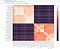Figure A: Corr Analysis Visualization using Seaborn Heatmap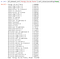Figure B: Correlation Analysis against Wind Energy (“Energy-ile-de-france”)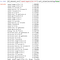Figure C: Corr Analysis against the Highest Corr Forecast in Figure B

# Data Analysis — Time Series: Detecting Trend (Seasonality)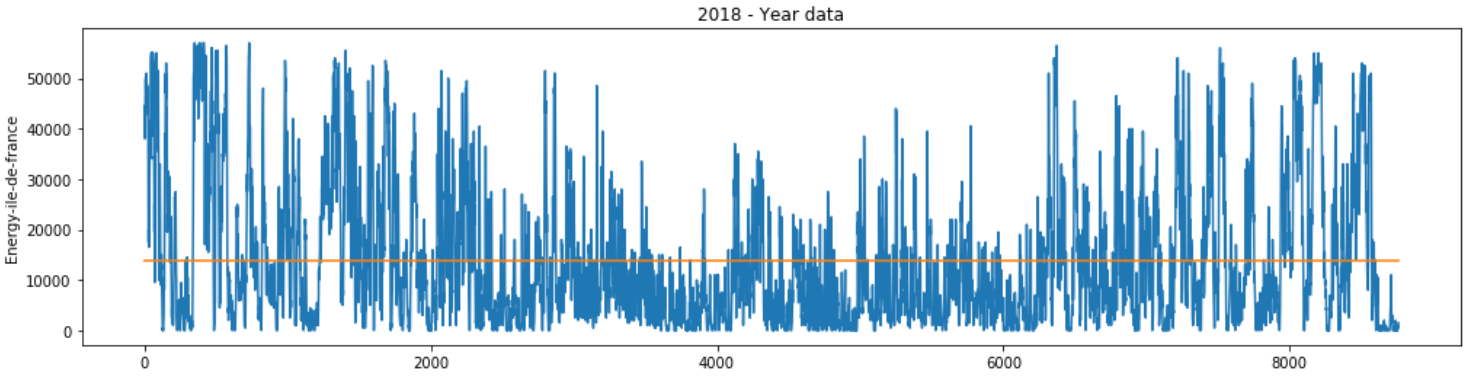Figure D(a): 2017 & 2018 Time Series Analysis for Wind Energy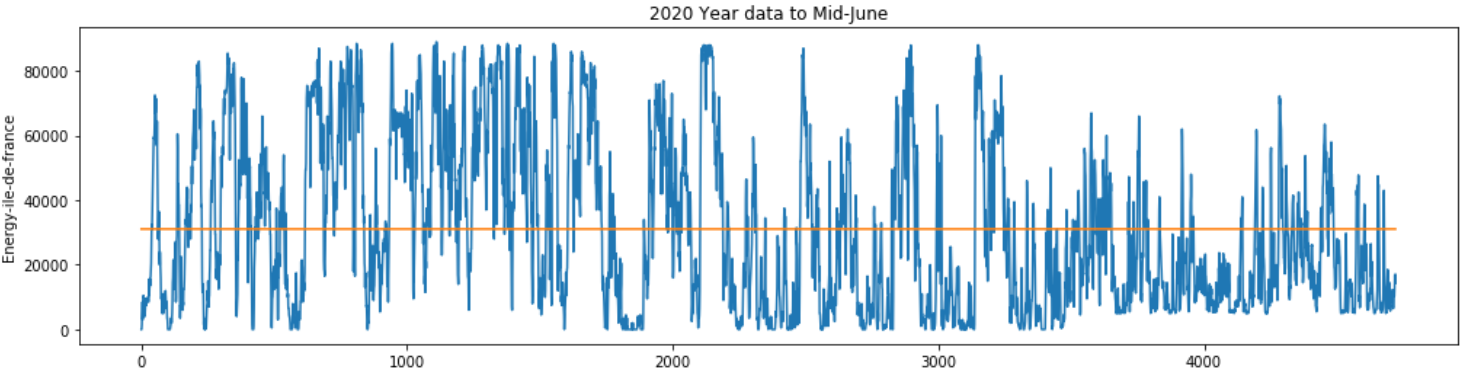Figure D(b): 2019 & 2020 Time Series Analysis for Wind Energy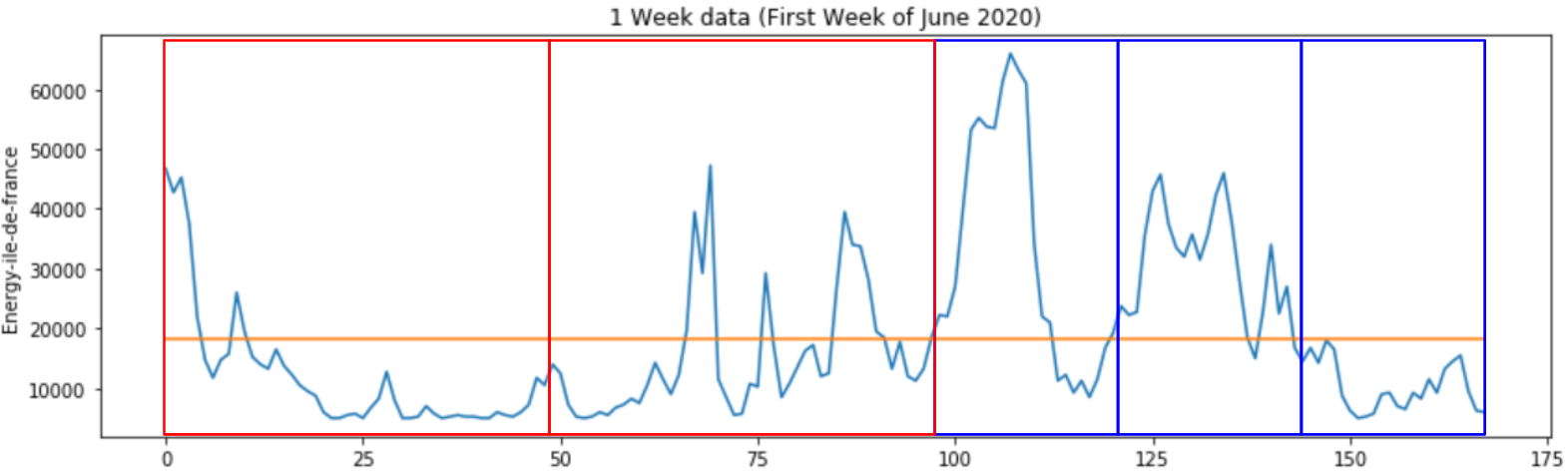Figure E: 1 Month & 1 WeekTime Series Analysis for Wind Energy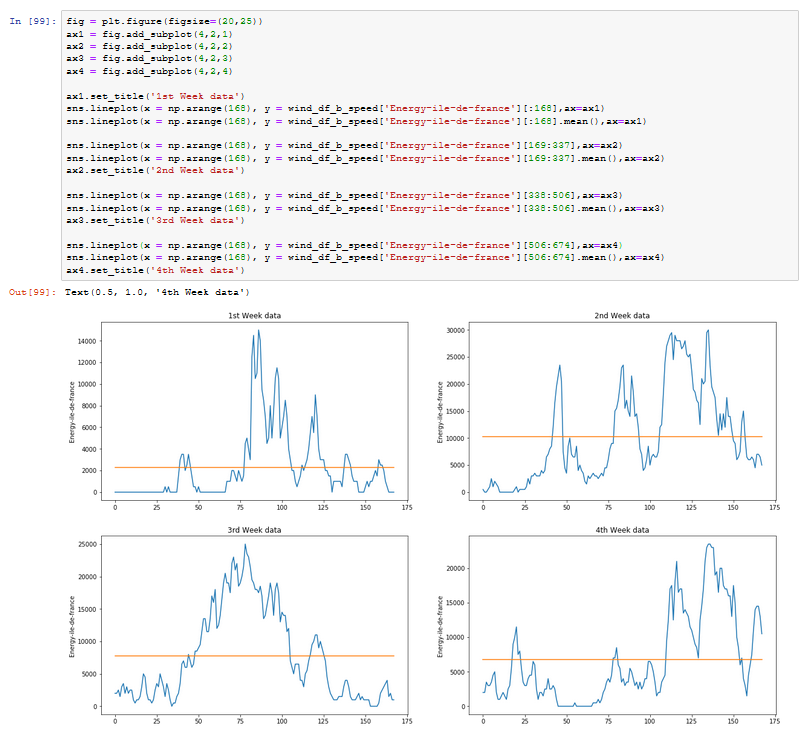Figure F(a): Weekly Time Series Analysis in 1 month (4 weeks)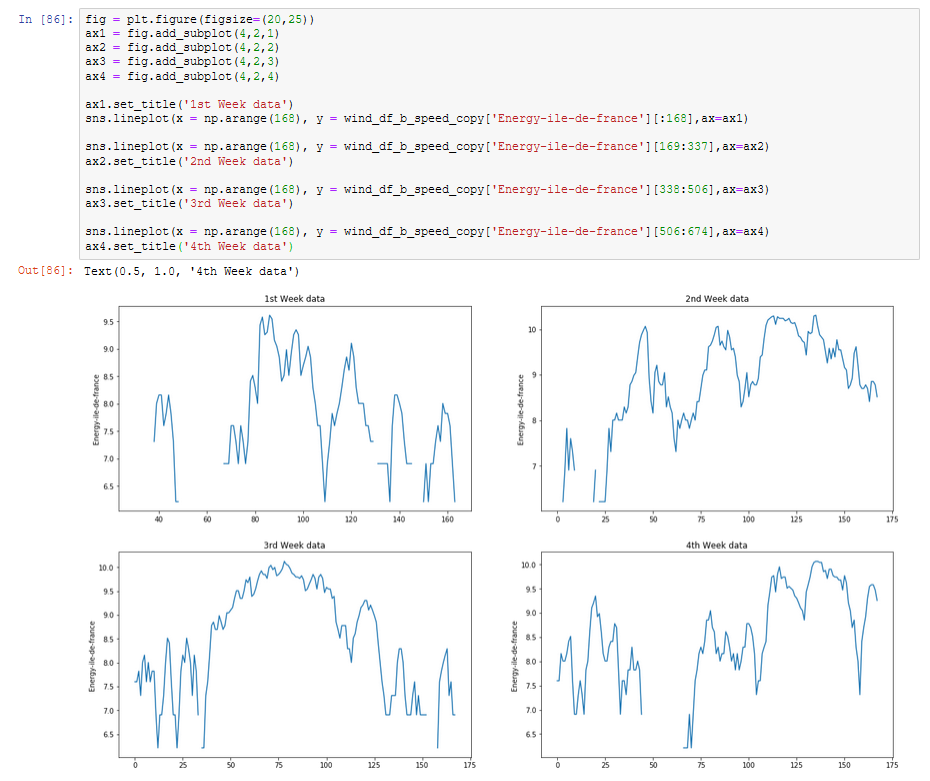Figure F(b): Weekly Time Series Analysis in 1 month (4 weeks-logarithmic scale)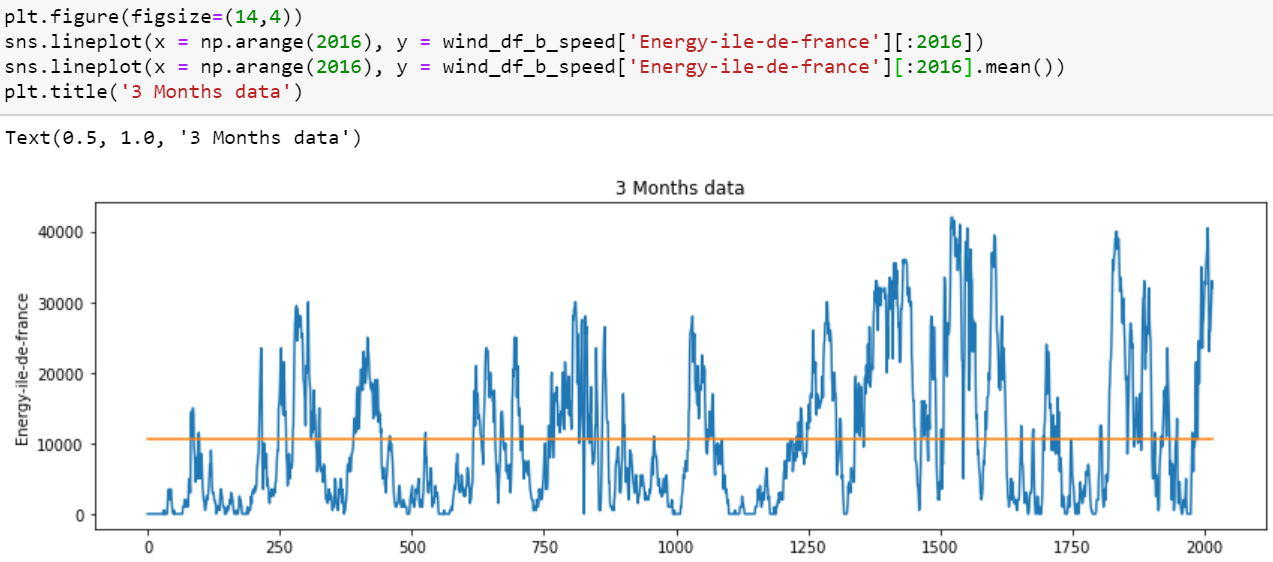Figure G(a) : 3 Months Time Series Analysis for Wind Energy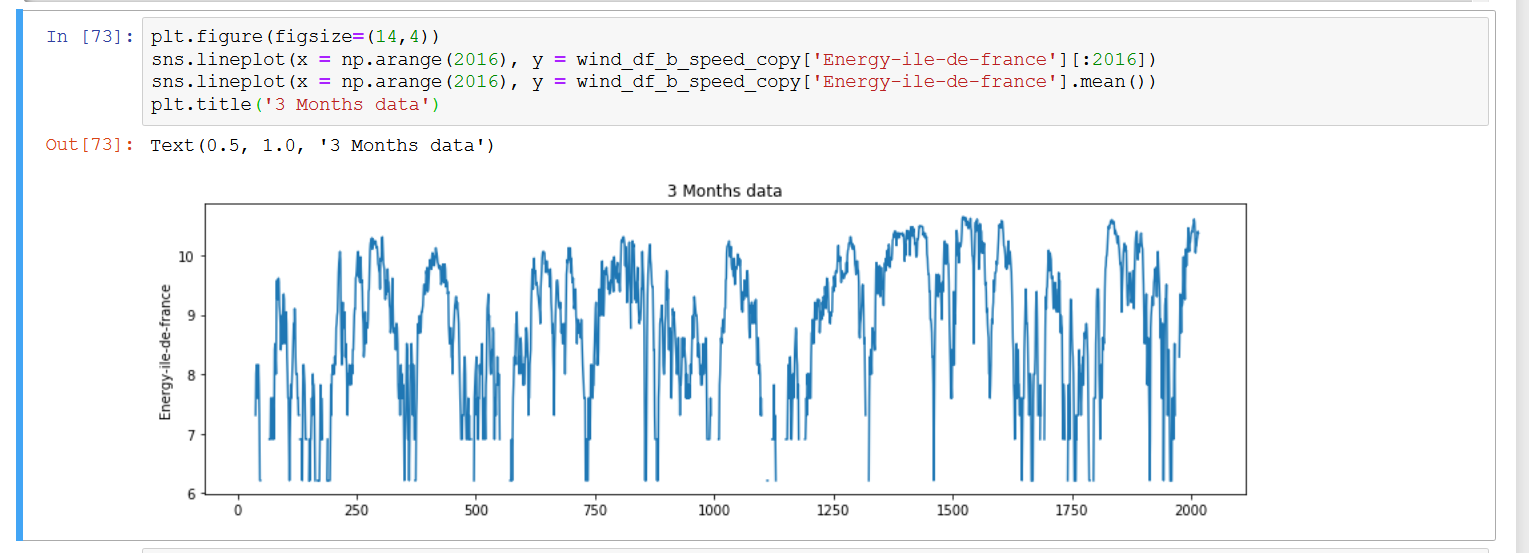Figure G(b): 3 Months Time Series Analysis for Wind Energy (logarithmic scale)

# Evaluation of Result for the First Trial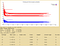Figure H: Training and Test Losses for First Trial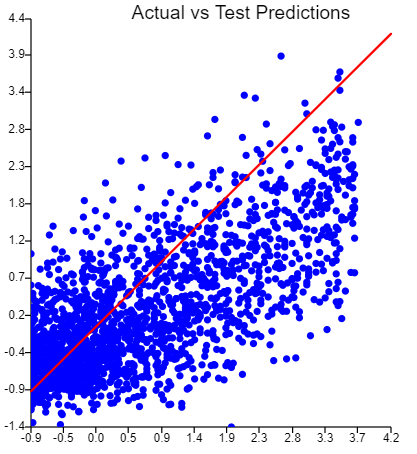Figure I: Actual vs Training / Test Prediction for First Trial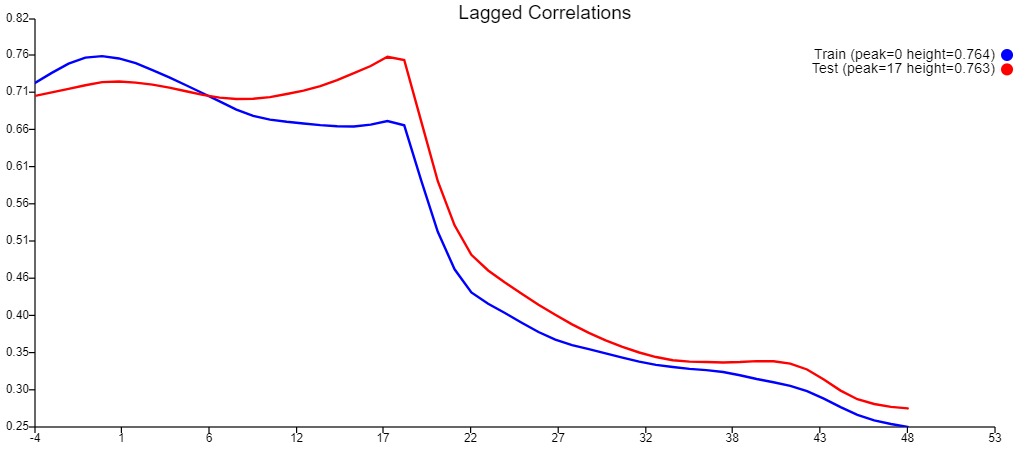Figure J: Lagged Correlations of First Trial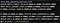Figure K: Profit from our First Trial Model

# Building Data Extractions (data.m)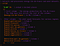Figure L: Data Extraction

# Feature Engineering (prep.m & pre.m)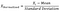Figure M: Normalizing formula

# Defining the Network (config.m & network.m)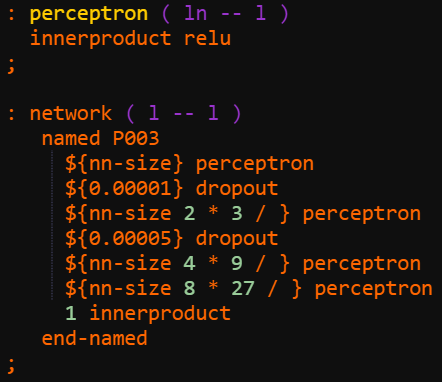Figure N: Our Network Layers

# Evaluation of our Model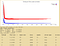Figure O: Graph of Training and Test Losses by iteration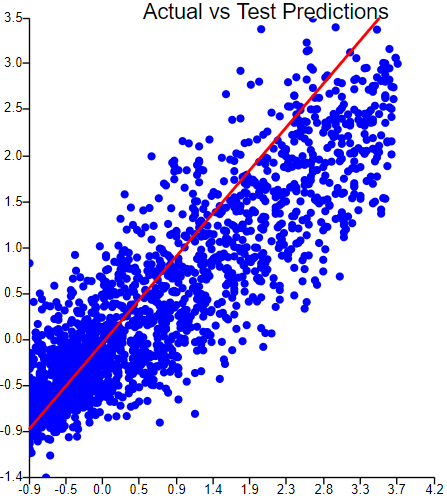Figure P: Actual vs Training / Test Prediction of Actual Model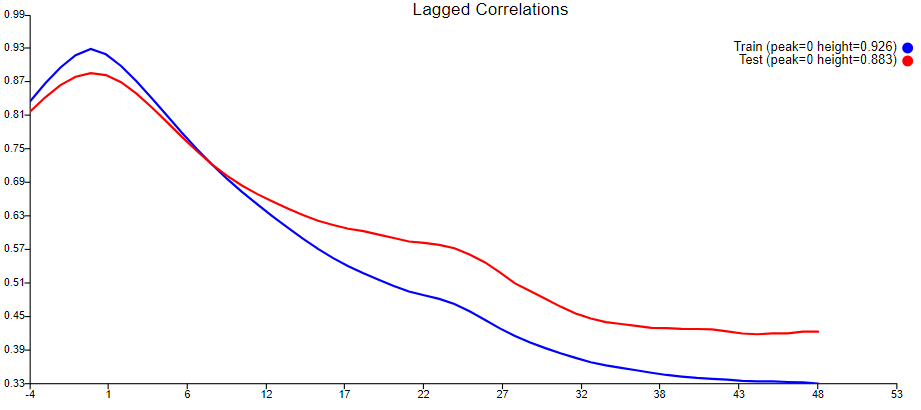Figure Q: Lagged Correlations of Actual Model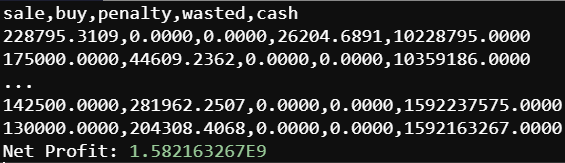Figure R: Net Profit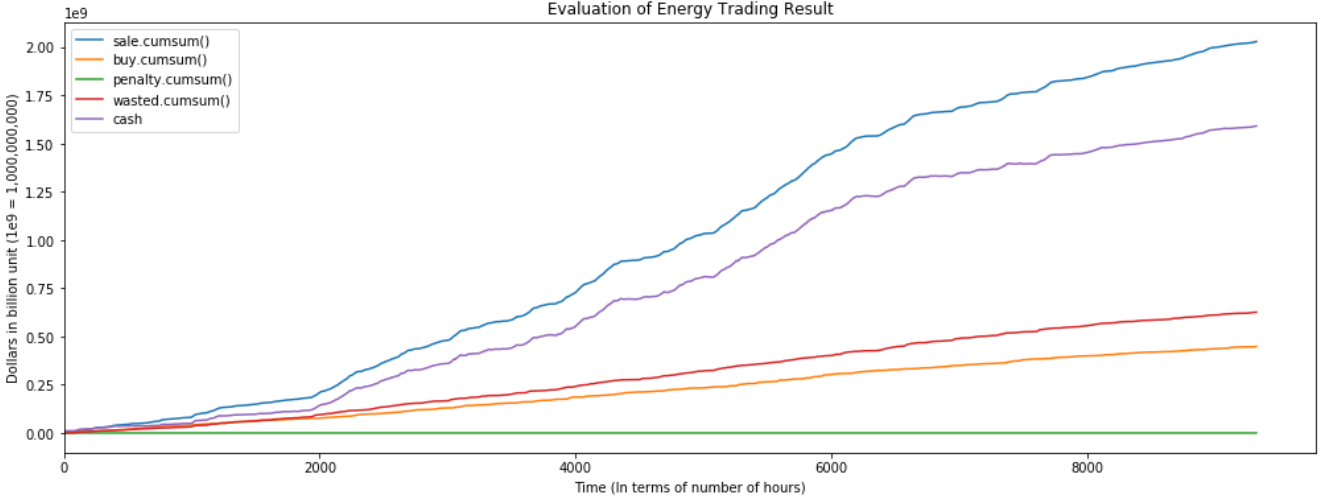Figure S: Earnings and Lost Time Series Graph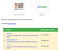Figure T: Live Deployment Result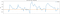Figure T: Live Deployment ROI

# Further Improvements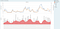Figure U: Time Series of Actual and Forecasted energy vs the Humidity and Weather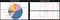Figure V: Weather and Humidity Data during those days that our model made a loss returns

Data Enthusiast